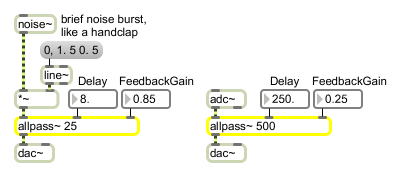# allpass~

Allpass filter

## Description

allpass~ implements the following filter:

y[n] = (-g * x[n]) + x[n - d] + (g * y[n - d])

where y is the output, x is the input, g is the gain (right inlet) and d is the delay (middle inlet) in milliseconds. The allpass has a flat frequency response but a complex phase response, typically delaying sharp transients. The gain (0-1) is a feedback coefficient that affects the recirculation of the delays.

## Arguments

Name Type Opt Description
filter-settings float opt Up to four numbers, to set the maximum delay time and initial values for the delay time D and gain coefficient g. If a signal is connected to a given inlet, the coefficient supplied as an argument for that inlet is ignored. If no arguments are present, the maximum delay time defaults to 10 milliseconds.

## Messages

 float filter-parameter [float] The filter parameters in the middle and right inlets may be specified by a float or int instead of a signal. If a signal is also connected to the inlet, the float or int is ignored. clear Clears the allpass~ object's memory of previous outputs, resetting them to 0. signal In left inlet: Any signal to be filtered. The filter mixes the current input sample with an earlier output sample, according to the formula: yn = -gxn + xn-(DR/1000) +gyn-(DR/1000) where R is the sampling rate and D is a delay time in milliseconds. In middle inlet: Delay time (D) in milliseconds for a past output sample to be added into the current output. In right inlet: Gain coefficient (g), for scaling the amount of the input and output samples to be sent to the output.

## Output

signal: The filtered signal.

## ExamplesShort delay with feedback to blur the input sound or longer delay for discrete echoes